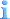# Guide: How to calculate park value?

## Recommended Posts

Park value is an important number for the completion of several scenarios. Many players do not fully understand how park value works, and struggle to complete those scenarios which require a high park value. When you know how park value works, those scenarios will actually become very easy.

This guide is divided in three chapters:
1. Park value formula
2. Ride value formula
3. Conclusions (tips)

If you dont like reading through code, you should probably skip to the third chapter, where I will give some tips on manipulating park value.

1. Park value formula

Park value is calculated in Park.cpp , in the function calculate_park_value().This function is actually quite simple. First, the program cycles through all rides and calculates their value. After that it adds €7.00 (I'll use € in this guide) for every guest in the park. So if the total value of your rides would be €100000 and you have 5000 guests in your park, the total park value would become €135000. Now, the difficult part of this function is calculating the ride value.

2. Ride value formula

To get the park value, the value of all rides has to be calculated. The formula to calculate ride value is as follows:There are three important variables in this function:
1. Ride->value
2. Ride customers in last 5 minutes
3. Ride bonus value

1. Ride value
Ride->value is calculated by taking the excitement, intensity and nausea of a ride, and multiplying those by certain factors. Then after that, the result is multiplied by an aging factor. Basically, the older the ride gets, the lower the value will be. In this guide, i have explained how to calculate this value, as it also determines how much you can charge for your ride!
The cool thing is that this part of the formula can be abused. If you make a ride with ultra-extreme intensity and nausea, and because Ride->value is for a large part a multiplication of the stats with a certain factor, this means that the ride value will become very high!

2. Ride customers in last 5 minutes
This part is quite self-explanatory. For a good ride value, you'll want rides with a high throughput of guests. If you need a high park rating, try building rides with a high capacity.

3. Ride bonus value
This is a bonus value for every ride, which is also used to calculate how many guests spawn in your park. The ride bonus values can be found in RideData.cpp, under const uint8 rideBonusValue[RIDE_TYPE_COUNT].Every ride type has its own bonus value.

Example

Now let's take an example ride to calculate its contribution to the park value. In the guide where I explained how much you can charge for your ride, we had an example Giga coaster which had an excitement of 8.00, an intensity of 5.50, and a nausea rating of 4.00. When we used the spreadsheet from that guide, we found a value of 88 when the ride was younger than 5 months. 88 will be the value we use for the Ride->value in the formula.For the number of riders in the last 5 minutes, lets just assume the ride has 1200 guests per hour, which gives us 100 guests in the last 5 minutes.

The ride bonus value of a Giga coaster can be looked up in the table in RideData.cpp, and it turns out the value is 120.We can now substitute those values in our formula:ride->value = 88,
ride_customers_in_last_5_minutes = 100,
rideBonusValue[ride->type] = 120

(88 * 10) * (100 + 120*4) = 510400

To get the contribution to the park value in money, this value has to be divided by 10, so this giga coaster would contribute €51400 to the park value.

This value will diminish over time as the ride gets older, because the ride->value component will get smaller over time. Also, it will be 25% less if there is already another ride of the same type in the park.

3. Conclusion (tips):

As explained in the previous chapters, the park value is calculated by summing the value of all rides, and then adding €7 to the value for every guest.

Here are some park value tips and considerations:

- For a high ride value, the stats of a ride need to be high. You can abuse this by making rides with extreme stats. A few very high roto-drop towers or freefall towers are a very cost-effective way of increasing your park rating.

- Build rides with high capacity and keep the throughput high.

- If rides break down, the throughput will be temporarily reduced, decreasing the park value. Try to keep downtime for rides low.

- Rain will also decrease the throughput for most rides, thereby temporarily decreasing the park value.

- If you build more than one of a ride type, their values will be reduced by 25%.

This guide used the soure code of OpenRCT2 at 21-03-2018.

•1